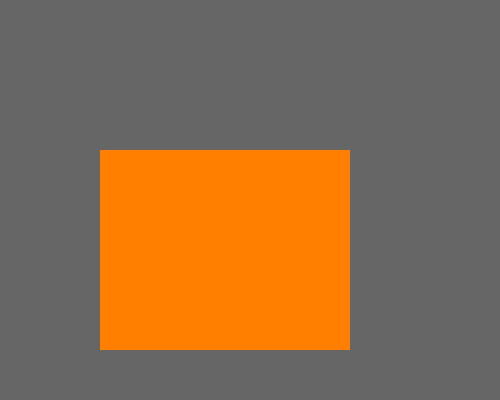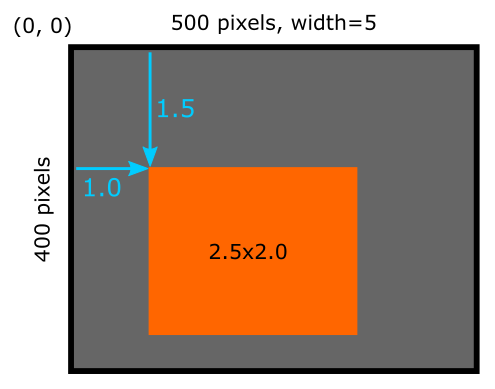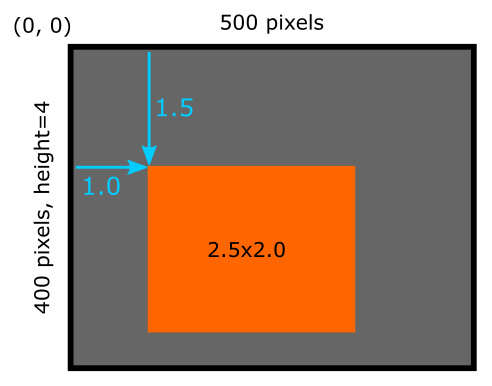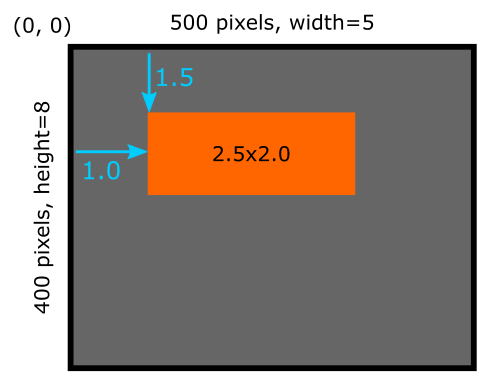# The setup function in generativepy

Martin McBride, 2021-11-07
Tags generativepy tutorial setup scaling
Categories generativepy generativepy tutorialIn this article we will see how to use the setup function generativepy.

## Previous example

In the previous article, simple image, we used this code:

from generativepy.drawing import make_image, setup
from generativepy.color import Color
from generativepy.geometry import Rectangle

def draw(ctx, pixel_width, pixel_height, frame_no, frame_count):

setup(ctx, pixel_width, pixel_height, background=Color(0.4))

color = Color(1, 0.5, 0)

Rectangle(ctx).of_corner_size((100, 150), 250, 200).fill(color)

make_image("rectangle.png", draw, 500, 400)


This created the following image:## Simple scaling

We can the setup function to scale the drawing space, simply by adding a width parameter:

from generativepy.drawing import make_image, setup
from generativepy.color import Color
from generativepy.geometry import Rectangle

def draw(ctx, pixel_width, pixel_height, frame_no, frame_count):

setup(ctx, pixel_width, pixel_height, width=5, background=Color(0.4))

color = Color(1, 0.5, 0)

Rectangle(ctx).of_corner_size((1.0, 1.5), 2.5, 2.0).fill(color)

make_image("rectangle.png", draw, 500, 400)


When we set width to 5 in the setup function it rescales our drawing coordinates so that values 0 to 5 map onto pixels 0 to 500. In other words, if we draw a rectangle that is 2.5 units wide, it will appear 250 pixels wide in the output image.

This code is available on github in tutorial/getting-started/setup.py.

When you set the width, setup will automatically scale the height too. Since our image is 400 pixels high, our user coordinates will be 4 units high (the same scaling factor, 1 unit is 100 pixels).

This code is available on github in tutorial/getting-started/setup.py.

Notice that we have changed the Rectangle code. It was previously:

Rectangle(ctx).of_corner_size((100, 150), 250, 200).fill(color)


We have divided the start coordinates and the width and height by 100 to adjust for the scaling factor. This code will create the exact same image as the previous example.

Here is a diagram showing the image with the new scaling:## Benefits of scaling

There are two benefits of scaling.

For one, it is sometimes convenient to be able to express the drawing code in particular units. For example, if you were creating a fractal that naturally occupies the unit square, you can use this technique to ensure that the unit square fills your image that might be 1000 pixels square. The alternative would be to do the conversions in your drawing code, which is possible but a little messy.

The second advantage is a bit more subtle. When you set the drawing space width to 5, then your drawing code can work to that size regardless of the pixel size of the image.

So, imagine you have created a really nice 500 by 400 pixel image. But you would like to create the same image at a higher resolution to print an art poster. All you need to do is change the size in make_image to, for example, 5000 by 4000 pixels. This will create a much higher resolution image, with every part of the image scaled up by a factor of 10. You would not need to change the draw function in any way to create the new image.

## Height scaling

We used this code to scale the drawing space down by 100:

setup(ctx, pixel_width, pixel_height, width=5, background=Color(0.4))


We could have done the same thing by setting a drawing space height instead:

setup(ctx, pixel_width, pixel_height, height=4, background=Color(0.4))


Since the pixel height is 400, setting the drawing space height to 400 creates a scale factor of 100, the same as when we set the width to 5. The image is identical:## Width and height scaling

This is not a feature that you will necessarily need to use very often, but we will look at it here just in case you might find it useful.

You can scale the width and height. This is particularly useful if you want to apply different scale factors in the x and y directions, like this:

setup(ctx, pixel_width, pixel_height, width=5, height=8, background=Color(0.4))


Here we are scaling the width of 500 pixels to a drawing width of 5 units (a scale factor of 100). We are also scaling the height of 400 pixels to a drawing height of 8 units (a scale factor of 50). This will distort the image like this:## Start positions

The setup function can also specify x and y start positions. Here is an example:

setup(ctx, pixel_width, pixel_height, width=5, startx=-1, starty=1, background=Color(0.4))


In this case, the top left-hand corner of the image no longer represents the origin, (0, 0). Instead, the origin now represents the position (-1, 1). This has the effect of shifting the drawing space by 1 unit to the right and 1 unit up:One particular use of this is to set up a drawing space where the origin is right in the centre of the image. To do this:

• Set startx to -width/2 where width is the width of the image drawing space.
• Set starty to -height/2 where height` is the height of the image drawing space.
If you found this article useful, you might be interested in the book Computer Graphics in Python or other books by the same author.

#### Popular tags

2d arrays abstract data type alignment and angle animation arc array arrays bar chart bar style behavioural pattern bezier curve built-in function callable object chain circle classes clipping close closure cmyk colour combinations comparison operator comprehension context context manager conversion count creational pattern data science data types decorator design pattern device space dictionary drawing duck typing efficiency ellipse else encryption enumerate fill filter font font style for loop function function composition function plot functools game development generativepy tutorial generator geometry gif global variable gradient greyscale higher order function hsl html image image processing imagesurface immutable object in operator index inner function input installing iter iterable iterator itertools join l system lambda function len lerp line line plot line style linear gradient linspace list list comprehension logical operator lru_cache magic method mandelbrot mandelbrot set map marker style matplotlib monad mutability named parameter numeric python numpy object open operator optimisation optional parameter or pandas partial application path pattern permutations pie chart polygon positional parameter print pure function python standard library radial gradient range recipes rectangle recursion reduce regular polygon repeat rgb rotation roundrect scaling scatter plot scipy sector segment sequence setup shape singleton slice slicing sound spirograph sprite square str stream string stroke structural pattern subpath symmetric encryption template text text metrics tinkerbell fractal transform translation transparency triangle truthy value tuple turtle unpacking user space vectorisation webserver website while loop zip